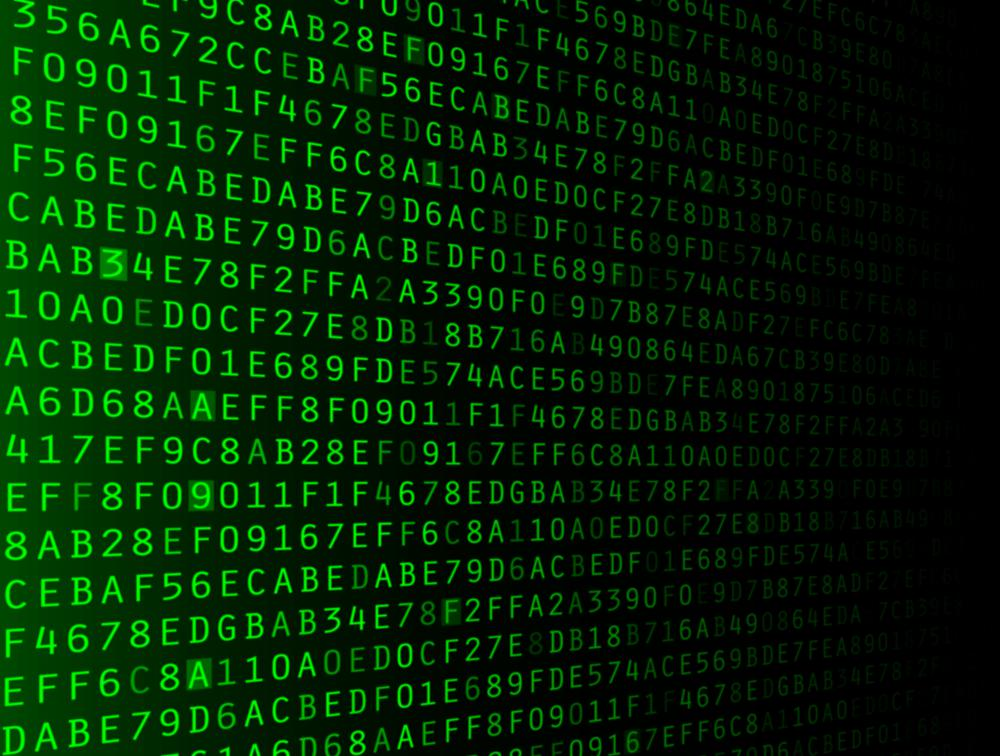# What Is an Associative Array?

Eugene P.

An associative array, also called a hash table or hash map, is similar to a standard array except the index of the array can be a string instead of an integer. In many database applications and in other programs that deal with large amounts of data, an associative array is a vital element in helping to sort and access information in an efficient way. At the core of an associative array is a standard array that is indexed with integers, as would normally be the case. A special algorithm called a hash function converts the string index into an integer index to find the value. This is a consistent conversion, so the actual integer index never needs to be stored but is instead calculated from the string as needed each time.A special algorithm called a hash function converts the string index into an integer index to find the value.

The terminology used when referring to an associative array can be slightly different than what is used when talking about a normal array. What would normally be called an index — the numerical location of an element inside an array — is called the key. The data associated with the key is called the value. This means that, within an associative array, a key is associated with a value, which correlates to an index referencing an element in a standard array in the data structure.

At the heart of every associative array is the hash function. This is an algorithm used to determine the numerical index of a value based on the key. There are several types of hash functions, some designed to operate on keys that are integers and some designed to work on keys that are strings. In the case of an integer key, a popular method is to divide the key value by the size of the array and use the remainder of the division to, hopefully, get a unique index value.Get started

The hash function can be much more complex for keys that are strings. Some methods include adding the numerical value of each character in the string and then dividing it by some number, or using only the first few characters of the string to get a unique number. There are many ways to derive a number from a string of characters.

When dealing with a large amount of key-value pairs in an associative array, one problem that can arise is called collision. Collision happens when the integer index derived from a key is identical to the integer index of another key. These two keys then effectively point to the same index in the value array. There are various solutions to collision, mainly because it has a high probability of happening in most practical applications.

One solution to collision is to have each value index actually be a linked list so that, when more than one key resolves to that index location, the location can hold more than one value. This is called chaining and is a simple way of handling a collision, though it also can slow down the time it takes to retrieve the information. Another method of dealing with a collision is called linear probing. When a collision occurs, linear probing works by moving through the value array until an unused index is found. This solution can help to keep the data evenly distributed in the associative array, but it also can increase the amount of time required to look up a value.